# Introduction

In the Eriksen flanker task participants are presented with an image of an odd number of arrows (usually five or seven). Their task is to indicate the orientation (left or right) of the middle arrow as quickly as possible whilst ignoring the flanking arrows on left and right. There are two types of stimuli in the task: in the congruent condition (e.g. ‘<<<<<<<‘) both the middle arrow and the flanking arrows point in the same direction, whereas in the incongruent condition (e.g. ‘<<<><<<‘) the middle arrow points to the opposite direction of the flanking arrows.

As the participants have to consciously ignore and inhibit the misleading information provided by the flanking arrows in the incongruent condition, the performance in the incongruent condition is robustly worse than in the congruent condition, both in terms of longer reaction times as well as higher proportion of errors. The difference between reaction times and error rates in congruent and incongruent cases is a measure of subject’s ability to focus his or her attention and inhibit distracting stimuli.

In the illustration below we compare reaction times and error rates when solving the flanker task between the control group (healthy subjects) and the test group (subjects suffering from a certain medical condition).

First, we load package bayes4psy and package dplyr for data wrangling. Second, we load the data and split them into control and test groups. For reaction time analysis we use only data where the response to the stimuli was correct:

# libs
library(bayes4psy)
library(dplyr)

data <- flanker

control_rt <- data %>% filter(result == "correct" &
group == "control")

test_rt <- data %>% filter(result == "correct" &
group == "test")

The model requires subjects to be indexed from $$1$$ to $$n$$. Control group subject indexes range from 22 to 45, so we cast it to 1 to 23.

# control group subject indexes range is 22..45 cast it to 1..23
# test group indexes are OK
control_rt$subject <- control_rt$subject - 21

Now we are ready to fit the Bayesian reaction time model for both groups. The model function requires two parameters – a vector of reaction times $$t$$ and the vector of subject indexes $$s$$. Note here that, due to vignette limitations, all fits are built using only one chain, using more chains in parallel is usually more efficient. Also to increase the building speed of vignettes we greatly reduced the amount of iterations, use an appropriate amount of iterations when executing actual analyses!

# prior
uniform_prior <- b_prior(family="uniform", pars=c(0, 3))

# attach priors to relevant parameters
priors <- list(c("mu_m", uniform_prior))

# fit
rt_control_fit <- b_reaction_time(t=control_rt$rt, s=control_rt$subject,
priors=priors,
chains=1, iter=200, warmup=100)

rt_test_fit <- b_reaction_time(t=test_rt$rt, s=test_rt$subject,
priors=priors,
chains=1, iter=200, warmup=100)

Before we interpret the results, we check MCMC diagnostics and model fit.

# plot trace
plot_trace(rt_control_fit)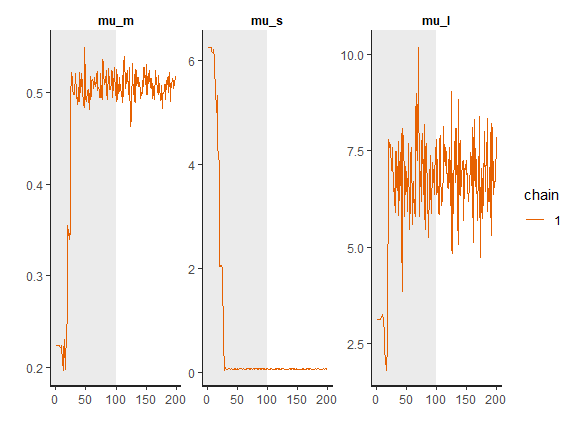plot_trace(rt_test_fit)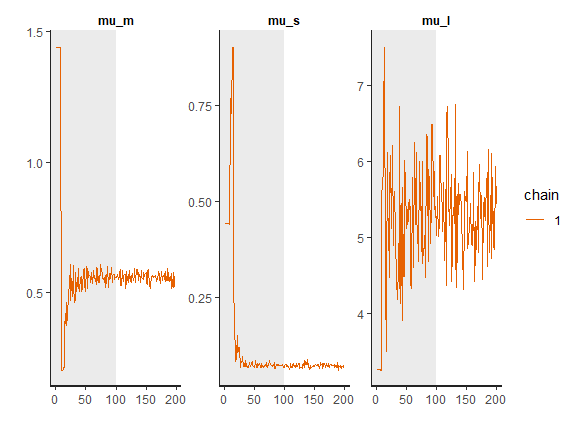The traceplots gives us no cause for concern regarding MCMC convergence and mixing. Note that the first 1000 iterations (shaded gray) are used for warmup (tuning of the MCMC algorithm) and are discarded. The next 1000 iterations are used for sampling.

# the commands below are commented out for the sake of brevity
#print(rt_control_fit)
#print(rt_test_fit)

The output above provides further MCMC diagnostics, which again do not give us cause for concern (only output of one fit is provided for the sake of brevity). The convergence diagnostic Rhat is practically 1 for all parameters and there is little auto-correlation (possibly even some positive auto-correlation) – effective sample sizes (n_eff) are of the order of samples taken and Monte Carlo standard errors (se_mean) are relatively small.

What is a good-enough effective sample sizes depends on our goal. If we are interested in posterior quantities such as the more extreme percentiles, the effective sample sizes should be 10,000 or higher, if possible. If we are only interested in estimating the mean, 100 effective samples is in most cases enough for a practically negligible Monte Carlo error.

We can increase the effective sample size by increasing the amount of MCMC iterations with the iter parameter. In our case we can achieve an effective sample size of 10,000 by setting iter to 4000. Because the MCMC diagnostics give us no reason for concern, we can leave the warmup parameter at its default value of 1000.

rt_control_fit <- b_reaction_time(t=control_rt$rt, s=control_rt$subject,
iter=4000)

rt_test_fit <- b_reaction_time(t=test_rt$rt, s=test_rt$subject,
iter=4000)

Because we did not explicitly define any priors, flat (improper) priors were put on all of the model’s parameters. In some cases, flat priors are a statement that we have no prior knowledge about the experiment results (in some sense). In general, even flat priors can express a preference for a certain region of parameter space. In practice, we will almost always have some prior information and we should incorporate it into the modelling process.

Next, we check whether the model fits the data well by using the plot function. If we set the subjects parameter to FALSE, we will get a less detailed group level fit. The data are visualized as a blue region while the fit is visualized with a black line. In this case the model fits the underlying data well.

# check fits
plot(rt_control_fit)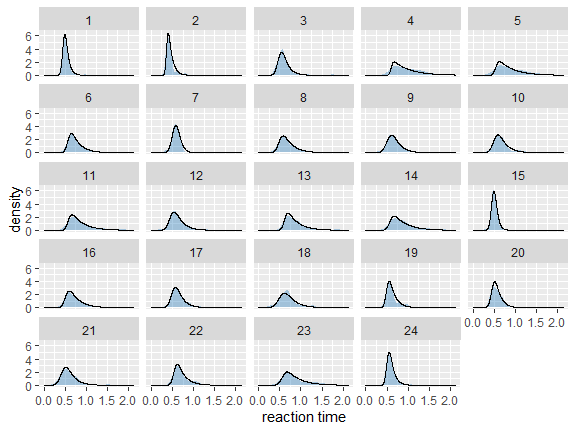plot(rt_test_fit)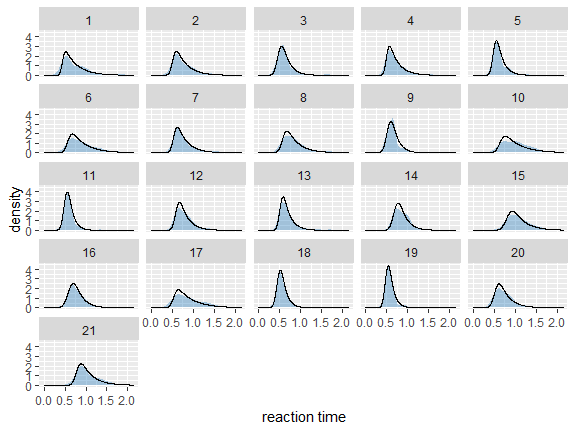We now use the compare_means function to compare reaction times between healthy (control) and unhealthy (test) subjects. In the example below we use a rope (region of practical equivalence) interval of 0.01 s, meaning that differences smaller that 1/100 of a second are deemed as equal. The compare_means function provides a user friendly output of the comparison and also returns the results in the form of a data.frame.

# set rope (region of practical equivalence) interval to +/- 10ms
rope <- 0.01

# which mean is smaller/larger
rt_control_test <- compare_means(rt_control_fit, fit2=rt_test_fit, rope=rope)
##
## ---------- Group 1 vs Group 2 ----------
## Probabilities:
##   - Group 1 < Group 2: 1.00 +/- 0.00000
##   - Group 1 > Group 2: 0.00 +/- 0.00000
##   - Equal: 0.00 +/- 0.00000
## 95% HDI:
##   - Group 1 - Group 2: [-0.15, -0.02]

The compare_means function output contains probabilities that one group has shorter reaction times than the other, the probability that both groups are equal (if rope interval is provided) and the 95% HDI (highest density interval) for the difference between groups. Based on the output we are quite certain (98% +/- 0.5%) that the healthy group’s (rt_control_fit) expected reaction times are lower than the unhealthy group’s (rt_test_fit).

We can also visualize this difference by using the plot_means_difference function. The plot_means function is an alternative for comparing rt_control_fit and rt_test_fit – the function visualizes the parameters that determine the means of each model.

# difference plot
plot_means_difference(rt_control_fit, fit2=rt_test_fit, rope=rope)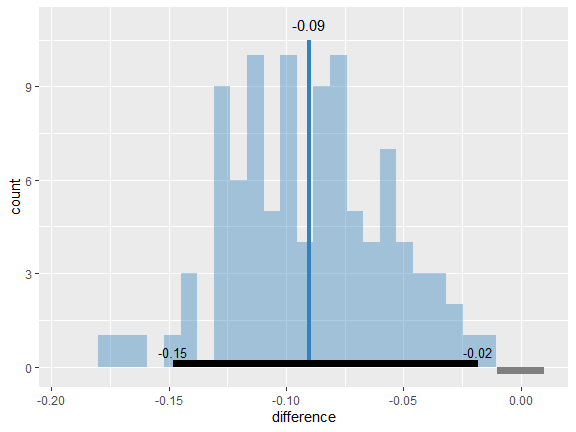The graph above visualizes the difference between rt_control_fit and rt_test_fit. The histogram visualizes the distribution of the difference, vertical blue line denotes the mean, the black band at the bottom marks the 95% HDI interval and the gray band marks the rope interval. Since the whole 95% HDI of difference is negative and lies outside of the rope interval we can conclude that the statement that healthy subjects are faster than unhealthy ones is most likely correct.

# visual comparsion of mean difference
plot_means(rt_control_fit, fit2=rt_test_fit)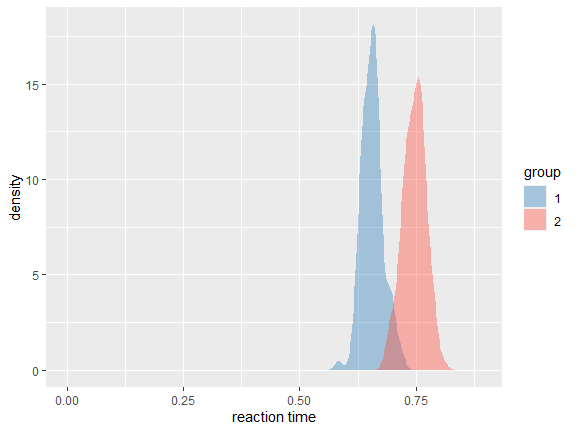Above you can see a visualization of means for rt_control_fit and rt_test_fit. Group 1 visualizes means for the healthy subjects and group 2 for the unhealthy subjects.

Based on our analysis we can claim with high probability (98% +/- 0.5%) that healthy subjects have faster reaction times when solving the flanker task than unhealthy subjects. Next, we analyze if the same applies to success rates.

The information about success of subject’s is stored as correct/incorrect. However, the Bayesian success rate model requires binary inputs (0/1) so we have to transform the data. Also, like in the reaction time example, we have to correct the indexes of control group subjects.

# map correct/incorrect/timeout to 1 (success) or 0 (fail)
data$result_numeric <- 0 data[data$result == "correct", ]$result_numeric <- 1 # split to control and test groups control_sr <- data %>% filter(group == "control") test_sr <- data %>% filter(group == "test") # control group subject indexes range is 22..45 cast it to 1..23 # test group indexes are OK control_sr$subject <- control_sr$subject - 21 Since the only prior information about the success rate of participants was the fact that success rate is located between 0 and 1, we used a beta distribution to put a uniform prior on the [0, 1] interval (we put a $$\BE(1, 1)$$ prior on the $$p$$ parameter). We execute the model fitting by running the b_success_rate function with appropriate input data. # priors p_prior <- b_prior(family="beta", pars=c(1, 1)) # attach priors to relevant parameters priors <- list(c("p", p_prior)) # fit sr_control_fit <- b_success_rate(r=control_sr$result_numeric,
s=control_sr$subject, priors=priors, chains=1, iter=200, warmup=100) ## ## SAMPLING FOR MODEL 'success_rate' NOW (CHAIN 1). ## Chain 1: ## Chain 1: Gradient evaluation took 0 seconds ## Chain 1: 1000 transitions using 10 leapfrog steps per transition would take 0 seconds. ## Chain 1: Adjust your expectations accordingly! ## Chain 1: ## Chain 1: ## Chain 1: WARNING: There aren't enough warmup iterations to fit the ## Chain 1: three stages of adaptation as currently configured. ## Chain 1: Reducing each adaptation stage to 15%/75%/10% of ## Chain 1: the given number of warmup iterations: ## Chain 1: init_buffer = 15 ## Chain 1: adapt_window = 75 ## Chain 1: term_buffer = 10 ## Chain 1: ## Chain 1: Iteration: 1 / 200 [ 0%] (Warmup) ## Chain 1: Iteration: 20 / 200 [ 10%] (Warmup) ## Chain 1: Iteration: 40 / 200 [ 20%] (Warmup) ## Chain 1: Iteration: 60 / 200 [ 30%] (Warmup) ## Chain 1: Iteration: 80 / 200 [ 40%] (Warmup) ## Chain 1: Iteration: 100 / 200 [ 50%] (Warmup) ## Chain 1: Iteration: 101 / 200 [ 50%] (Sampling) ## Chain 1: Iteration: 120 / 200 [ 60%] (Sampling) ## Chain 1: Iteration: 140 / 200 [ 70%] (Sampling) ## Chain 1: Iteration: 160 / 200 [ 80%] (Sampling) ## Chain 1: Iteration: 180 / 200 [ 90%] (Sampling) ## Chain 1: Iteration: 200 / 200 [100%] (Sampling) ## Chain 1: ## Chain 1: Elapsed Time: 0.771 seconds (Warm-up) ## Chain 1: 0.613 seconds (Sampling) ## Chain 1: 1.384 seconds (Total) ## Chain 1: sr_test_fit <- b_success_rate(r=test_sr$result_numeric,
s=test_sr\$subject,
priors=priors,
chains=1, iter=200, warmup=100)
##
## SAMPLING FOR MODEL 'success_rate' NOW (CHAIN 1).
## Chain 1:
## Chain 1: Gradient evaluation took 0.001 seconds
## Chain 1: 1000 transitions using 10 leapfrog steps per transition would take 10 seconds.
## Chain 1:
## Chain 1:
## Chain 1: WARNING: There aren't enough warmup iterations to fit the
## Chain 1:          three stages of adaptation as currently configured.
## Chain 1:          Reducing each adaptation stage to 15%/75%/10% of
## Chain 1:          the given number of warmup iterations:
## Chain 1:            init_buffer = 15
## Chain 1:            adapt_window = 75
## Chain 1:            term_buffer = 10
## Chain 1:
## Chain 1: Iteration:   1 / 200 [  0%]  (Warmup)
## Chain 1: Iteration:  20 / 200 [ 10%]  (Warmup)
## Chain 1: Iteration:  40 / 200 [ 20%]  (Warmup)
## Chain 1: Iteration:  60 / 200 [ 30%]  (Warmup)
## Chain 1: Iteration:  80 / 200 [ 40%]  (Warmup)
## Chain 1: Iteration: 100 / 200 [ 50%]  (Warmup)
## Chain 1: Iteration: 101 / 200 [ 50%]  (Sampling)
## Chain 1: Iteration: 120 / 200 [ 60%]  (Sampling)
## Chain 1: Iteration: 140 / 200 [ 70%]  (Sampling)
## Chain 1: Iteration: 160 / 200 [ 80%]  (Sampling)
## Chain 1: Iteration: 180 / 200 [ 90%]  (Sampling)
## Chain 1: Iteration: 200 / 200 [100%]  (Sampling)
## Chain 1:
## Chain 1:  Elapsed Time: 0.551 seconds (Warm-up)
## Chain 1:                0.572 seconds (Sampling)
## Chain 1:                1.123 seconds (Total)
## Chain 1:

The process for inspecting Bayesian fits is the same as above. When visually inspecting the quality of the fit (the plot function) we can set the subjects parameter to FALSE, which visualizes the fit on the group level. This offers a quicker, but less detailed method of inspection. Again one of the commands is commented out for the sake of brevity.

# inspect control group fit
plot_trace(sr_control_fit)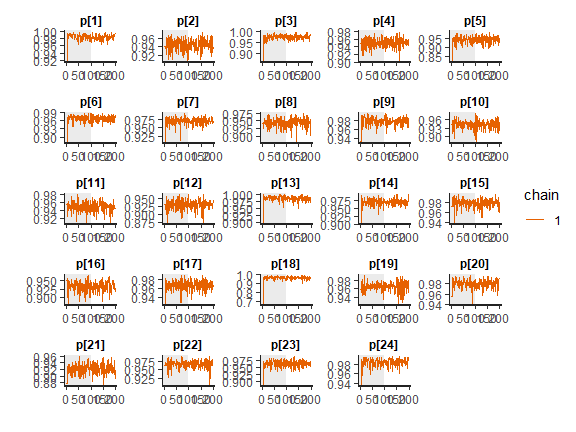plot(sr_control_fit, subjects=FALSE)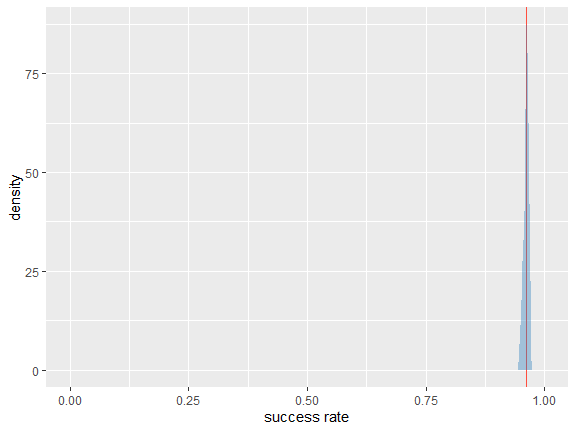print(sr_control_fit)
## Inference for Stan model: success_rate.
## 1 chains, each with iter=200; warmup=100; thin=1;
## post-warmup draws per chain=100, total post-warmup draws=100.
##
##          mean se_mean    sd    2.5%     25%     50%     75%   97.5% n_eff Rhat
## p0       0.96    0.00  0.00    0.95    0.96    0.96    0.96    0.97   154 0.99
## tau     85.42    5.74 38.34   35.61   57.39   77.48  107.62  162.42    45 1.02
## p     0.99    0.00  0.01    0.97    0.98    0.98    0.99    1.00    55 1.03
## p     0.95    0.00  0.01    0.92    0.94    0.95    0.95    0.97   112 1.01
## p     0.98    0.00  0.01    0.96    0.97    0.98    0.98    1.00    58 1.03
## p     0.95    0.00  0.01    0.93    0.94    0.95    0.96    0.97   146 1.00
## p     0.95    0.00  0.02    0.90    0.93    0.95    0.96    0.98   200 1.00
## p     0.97    0.00  0.01    0.94    0.96    0.97    0.97    0.98   200 0.99
## p     0.97    0.00  0.01    0.95    0.96    0.97    0.98    0.98   120 1.00
## p     0.94    0.00  0.02    0.91    0.93    0.95    0.95    0.97   156 0.99
## p     0.98    0.00  0.01    0.96    0.97    0.98    0.98    0.99   110 0.99
## p    0.94    0.00  0.02    0.91    0.93    0.94    0.95    0.97   200 0.99
## p    0.95    0.00  0.01    0.92    0.94    0.95    0.96    0.97   136 1.00
## p    0.93    0.00  0.01    0.90    0.92    0.93    0.94    0.95   126 0.99
## p    0.98    0.00  0.01    0.97    0.98    0.98    0.99    1.00    85 1.00
## p    0.97    0.00  0.01    0.95    0.97    0.97    0.98    0.99    77 0.99
## p    0.98    0.00  0.01    0.96    0.97    0.98    0.99    0.99   115 1.00
## p    0.93    0.00  0.01    0.91    0.92    0.93    0.94    0.96   117 1.00
## p    0.97    0.00  0.01    0.95    0.96    0.97    0.97    0.98   200 0.99
## p    0.96    0.00  0.02    0.92    0.95    0.96    0.97    0.98    98 1.00
## p    0.97    0.00  0.01    0.94    0.96    0.97    0.97    0.99   200 0.99
## p    0.98    0.00  0.01    0.96    0.97    0.98    0.99    0.99   126 1.01
## p    0.92    0.00  0.02    0.89    0.91    0.92    0.94    0.95   200 0.99
## p    0.97    0.00  0.01    0.94    0.96    0.97    0.98    0.98    78 0.99
## p    0.96    0.00  0.01    0.94    0.95    0.96    0.97    0.98   200 0.99
## p    0.98    0.00  0.01    0.97    0.98    0.99    0.99    1.00    98 1.01
## lp__  -708.25    0.76  4.55 -716.39 -711.38 -708.74 -705.14 -700.11    36 1.00
##
## Samples were drawn using NUTS(diag_e) at Mon Sep 27 10:54:28 2021.
## For each parameter, n_eff is a crude measure of effective sample size,
## and Rhat is the potential scale reduction factor on split chains (at
## convergence, Rhat=1).
# inspect test group fit
plot_trace(sr_test_fit)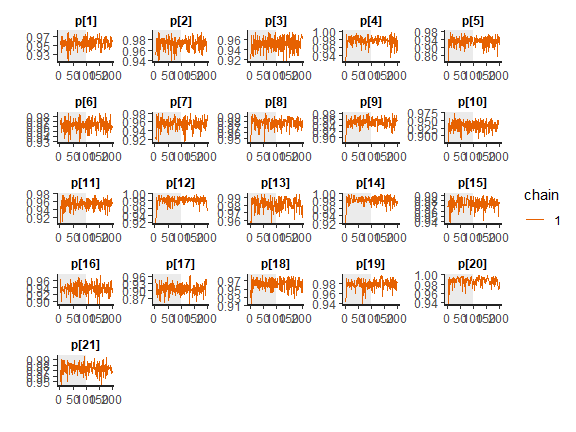plot(sr_test_fit, subjects=FALSE)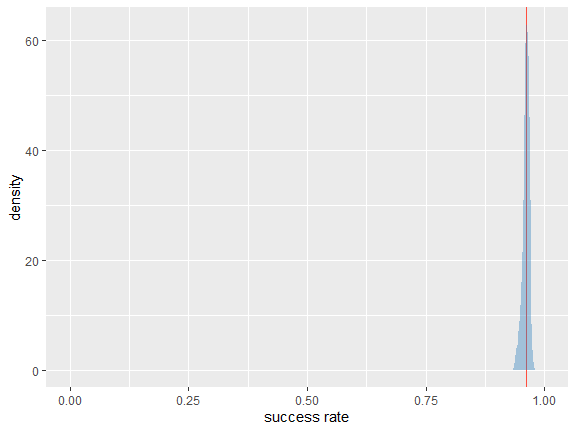#print(sr_test_fit)

Since diagnostic functions show no pressing issues and the fits look good we can proceed with the actual comparison between the two fitted models. We will again estimate the difference between two groups with compare_means.

# which one is higher
sr_control_test <- compare_means(sr_control_fit, fit2=sr_test_fit)
##
## ---------- Group 1 vs Group 2 ----------
## Probabilities:
##   - Group 1 < Group 2: 0.51 +/- 0.05024
##   - Group 1 > Group 2: 0.49 +/- 0.05024
## 95% HDI:
##   - Group 1 - Group 2: [-0.01, 0.02]

As we can see the success rate between the two groups is not that different. Since the probability that healthy group is more successful is only 53% (+/- 1%) and the 95% HDI of the difference ([0.02, 0.02]) includes the 0 we cannot claim inequality. We can visualize this result by using the function.

# difference plot
plot_means_difference(sr_control_fit, fit2=sr_test_fit)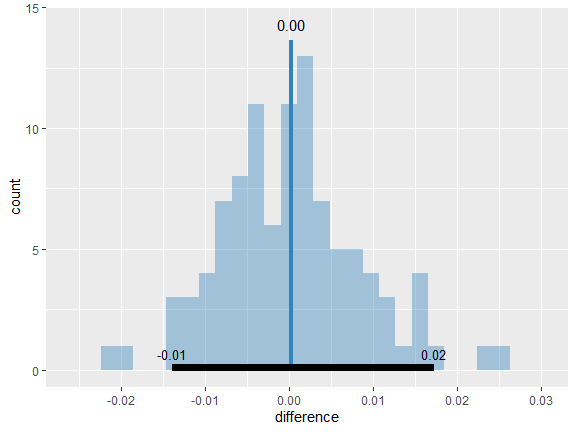Above you can see the visualization of the difference between the sr_control_fit and the sr_test_fit. Histogram visualizes the distribution of the difference, vertical blue line denotes the mean difference and the black band at the bottom marks the 95% HDI interval. Since the 95% HDI of difference includes the value of 0 we cannot claim inequality. If we used a rope interval and the whole rope interval lied in the 95% HDI interval we could claim equality.